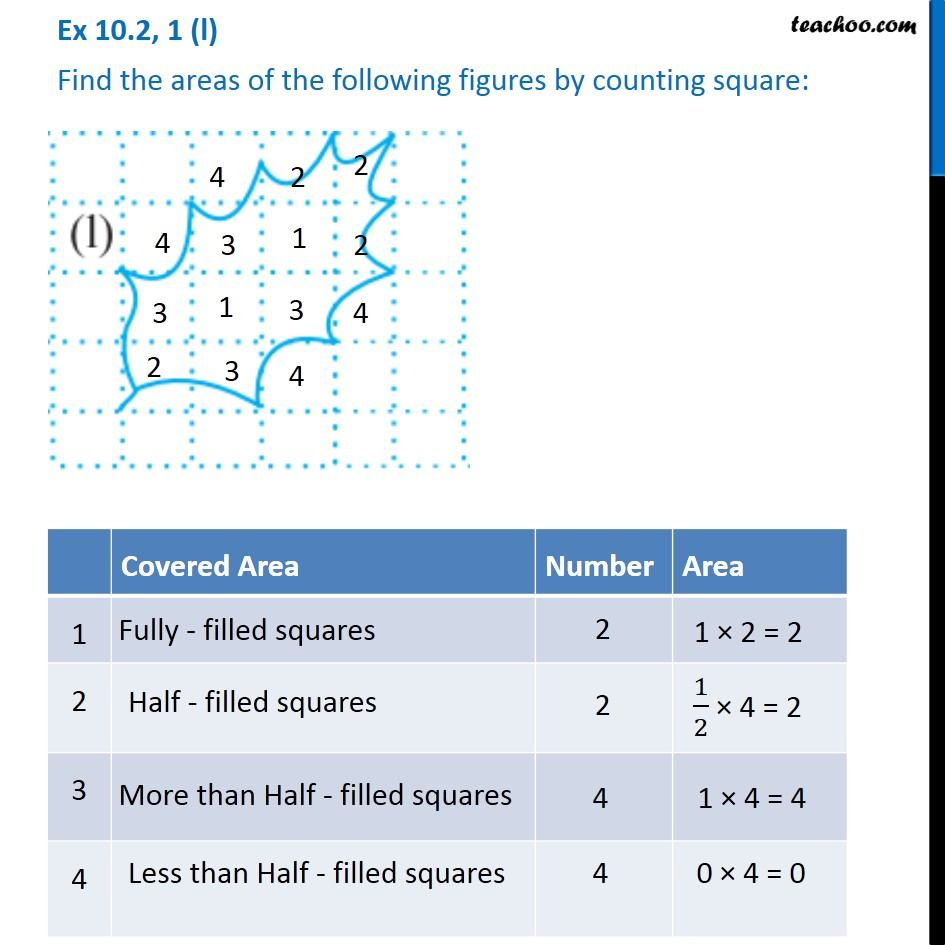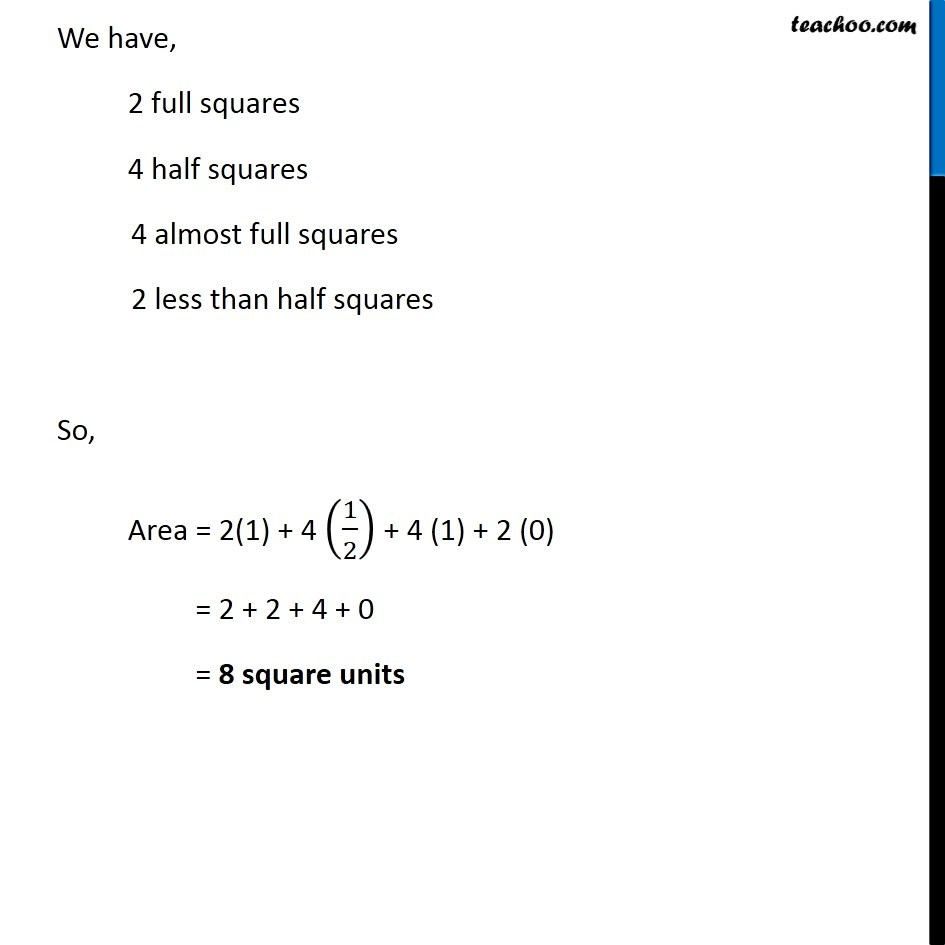1. Chapter 10 Class 6 Mensuration
2. Concept wise
3. Finding area by counting squares

Transcript

Ex 10.2, 1 (l) Find the areas of the following figures by counting square: Covered Area Number Area 1 Fully filled squares 2 1 × 2 = 2 2 Half filled squares 2 1/2 × 4 = 2 3 More than Half filled squares 4 1 × 4 = 16 4 Less than half filled squares 4 0 × 4 = 0 We have, 2 full squares 4 half squares 4 almost full squares 2 less than half squares So, Area = 2(1) + 4 (1/2) + 4 (1) + 2 (0) = 2 + 2 + 4 + 0 = 8 square units